# Train

The train passes part of the line for 95 minutes at speed 75 km/h. What speed would have to go in order to shorten the driving time of 20 minutes?

Correct result:

v =  95 km/h

#### Solution: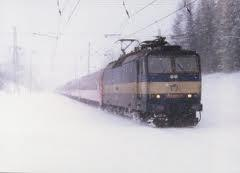We would be pleased if you find an error in the word problem, spelling mistakes, or inaccuracies and send it to us. Thank you!Tips to related online calculators
Check out our ratio calculator.
Do you want to convert velocity (speed) units?

## Next similar math problems:

• Compare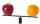Compare with characters >, <, =: 85.57 ? 80.83
• DoctorsIn the city operates 196 doctors. The city has 134456 citizens. How many citizens are per one doctor?
• Roman numeralsWrite numbers written in Roman numerals as decimal.
• To thousands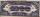Round to thousands following numbers:
• Roman numerals 2+Add up the number writtens in Roman numerals. Write the results as a roman numbers.
• ProductResult of the product of the numbers 1, 2, 3, 1, 2, 0 is:Added together and write as decimal number: LXVII + MLXIV
• Roman numerals +Add up the number writtens in Roman numerals. Write the results as a decimal number.
• Shaving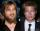Mathematical problem from Sunday evening. If the beard is growing at 0.52 mm per day and that my length is 16.8 mm, how long I don't shave?
• MedianThe median of the triangle LMN is away from vertex N 84 cm. Calculate the length of the median, which start at N.
• Rate 3A liquid is heated at a rate of 5 degrees Celsius per minute. If the temperature now is at 39 degrees Celsius, after how long will the temperature be 94 degrees Celsius?
• Speedometer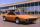The speedometer of a car reads 66 km/h. It's accurate to within 4.1% What is the maximum possible error?
• Avg speed of flight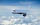The students vice adventure had a 2,367 km flight. If they travel time was 2 hours and 56 minutes, what was their average speed in kilometres per hour?
• Monthly payments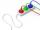Novákovi pay for electricity and monthly pays at the end of the year still bill 34 USD. Total per year pay 618 USD. What were their monthly payments?
• Cyclist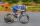A cyclist passes 88 km in 4 hours. How many kilometers he pass in 8 hours?
• Tourist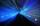Tourist went 24 km for 4 hours. How many meters he goes at the same speed for 12 minutes? ? Help - convert units to minutes and meters
• Trams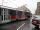Trams have an average speed 23 km/h and run in tact 14 minutes. Pedestrian walking speed is 3.3 km/h. At what intervals trams outrun pedestrian?